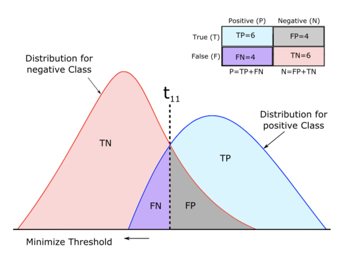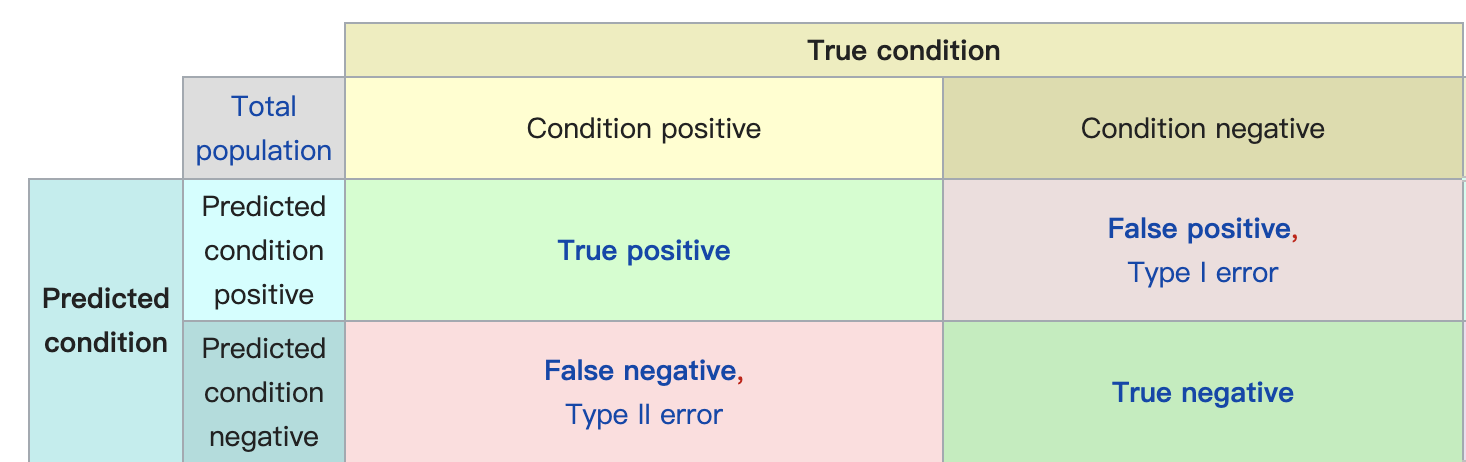# Binary classification - 聊聊评价指标的那些事儿【回忆篇】TP:预测为正&真实为正
FP:预测为正&真实为负
TN:预测为负&真实为负
FN:预测为负&真实为正

$Accuracy = \frac{TP + TN}{TP + TN + FN + FP}$

\begin{align} precision &= \frac{TP}{TP+FP} \\ recall &= \frac{TP}{TP+FN} \end{align}

$F1 =\frac{1}{\frac{1}{precision} + \frac{1}{recall}}= \frac{precision * recall}{precision + recall}$

sensitivity, sepcifity都从真实分布的角度，分别衡量正/负样本预测的准确率。这一对搭配最常在医学检验中出现，衡量实际生病/没生病的人分别被正确检验的概率。正确检验出一个人有病很重要，同时正确排除一个人没生病也很重要。

\begin{align} sensitivity &= recall \\ specifity & =\frac{TN}{TN + FP} \\ \end{align}

$fpr = \frac{FP}{TN+FP} = 1- specifity$

F1 score
https://www.kaggle.com/c/quora-insincere-questions-classification/overview/evaluation

accuracy
https://www.kaggle.com/c/titanic/overview/evaluation

tpr(recall) + fpr = ROC-> AUC